C Program to Calculate Bonus & Gross using Basic Salary

In this tutorial you will learn about the C Program to Calculate Bonus & Gross using Basic Salary and its application with practical example.

C Program to Calculate Bonus & Gross using Basic Salary

In this tutorial, we will learn to create a C program that will Calculate bonuses & Gross using Basic Salary using C programming.

Prerequisites

Before starting with the tutorial we assume that you are best aware of the following C programming topics:

• Operators in C Programming.
• Basic Input and Output function in C Programming.
• Basic C programming.
• Conditional statement in C programming.

Program to Calculate bonuses & Gross using Basic Salary

In c programming, it is possible to take numerical input from the user and calculate the bonus & Gross using Basic Salary with the help of a very small amount of code. The C language has many types of header libraries which has supported function in them with the help of these files the programming is easy.

Algorithm:-

With the help of this program, we can Calculate the bonus & Gross using Basic Salary.

Output:-

In the above program, we have first initialized the required variable.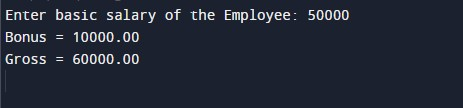• basic = it will hold the float value for the basic salary.
• bonus = it will hold the float value for the bonus salary.
• gross = it will hold the float value for the gross salary.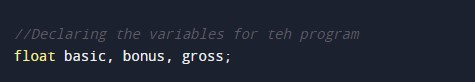Input salary for the employee.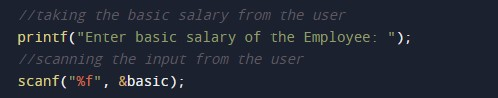Bonus Salary for the employee.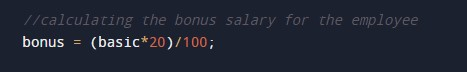Gross Salary for the employee.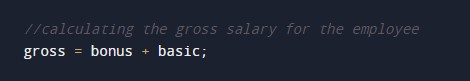Printing output for salary for the employee.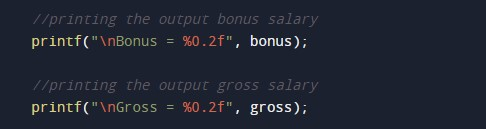In this tutorial we have learn about the C Program to Calculate Bonus & Gross using Basic Salary and its application with practical example. I hope you will like this tutorial.